# Volumetric Gas Flow Switches Information

Volumetric gas flow switches measure the flow or quantity of a moving gas in terms of a unit of volume per time, such as cubic meters per second (m3 s-1). These flow sensing devices have a switch output.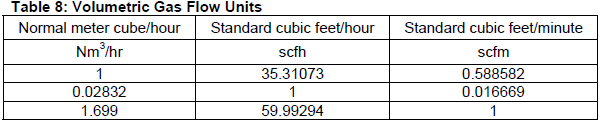Volumetric gas flow units. Table Credit: isa.org

Volume flow is commonly used to describe and quantify pumping speeds. Volume flow rate is defined by a limit. The equation below shows the flow of volume of fluid V through a surface unit time t. The change in volume is the amount of flow after crossing the boundary for some time duration.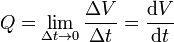This fundamental equation is derived from a simple equation which is only true for flat, plane cross-section. The surface integral is used for curved surfaces.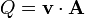Where:

v = velocity field of the substance element flowing

A = cross- section vector area/surface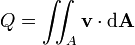Volumetric flow rate relates to mass flow rate, since mass relates to volume via density. Mass flow measures the mass or weight of the media flowing through a system. The units are typically given in weight per unit time. Mass does not change based on temperature or pressure, however volume does change and it is important to account for changes in system temperature and pressure. This can be done by knowing the density of the media throughout the process. The mass flow rate can be calculated from the volumetric flow rate using the equation below.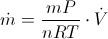This equation is derived from the ideal gas law PV = nRT and the equation for gas density ρ = m/V. These equations are then substituted into the equation for mass flow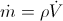.

## Pipe and Switch Specifications

Pipe size and pipe style are important specifications to consider when selecting volumetric gas flow switches. There are three main parameters: pipe diameter, mounting style, and end fittings.

• The pipe diameter describes the diameter of the process pipe to be monitored. Both the inner diameter (ID) and outer diameter (OD) are important to know to ensure that the flow switch fits securely onto the system. It is specified in English units such as inches (in) or metric units such as centimeters (cm).
• There are three choices for mounting type or style:
• In-line,
• Insertion
• Non-invasive.
• End fittings for volumetric gas flow switches include clamps as well as compression, flanged, plain end, threaded, and tube end fittings. Socket welds or unions are also used.

Switch specifications include:

• Switch type - Most devices have either solid state or electromechanical switches.
• Normal state - There are two normal states: normally open (NO) and normally closed (NC).

• Number of poles and throws - Volumetric liquid flow switches are also designated as single pole (SP) or double pole (DP), and single throw (ST) or double throw (DT).

More information on pipe and switch specifications can be found on GlobalSpec's How to Select Flow Switches.

## Meter Technology

Some volumetric gas flow switches are used with differential pressure (DP) meters or positive displacement (PD) meters.

### Differential Pressure Meters

With differential pressure meters, the basic operating principle is that the pressure drop across the meter is proportional to the square of the flow rate. The flow rate is obtained by measuring the pressure differential and extracting the square root. Differential pressure meters have a primary and secondary element. The primary element causes a change in kinetic energy, which creates the differential pressure in the pipe. The unit must be properly matched to the pipe size, flow conditions, and the liquid's properties. In addition, the measurement accuracy of the element must be accurate over a reasonable range. The secondary element measures the differential pressure and provides the signal or read-out that is converted to the actual flow value. DP meters include: orifice plates, venturi tubes, flow nozzles, cone types, pitot tubes, target meters, elbow tap meters, and rotameters.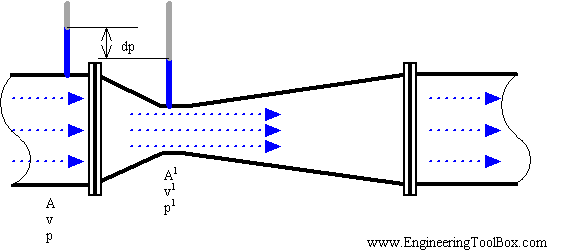Venturi tube. Image Credit: Engineering ToolBox

### Positive Displacement Meters

Positive displacement meters are used to take direct measurements of liquid flow. PD meters divide the liquid into specific increments which are moved on. The total flow is an accumulation of the measured increments. Typically, volumetric liquid flow switches count these increments by mechanical or electronic techniques. Positive displacement flow meters are often used for high-viscosity fluids.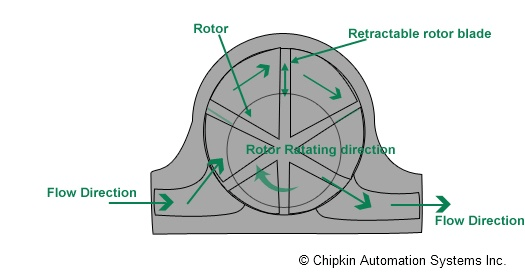Rotary-vane meter. Image Credit: Chipkin Automation System Inc.

### Velocity Meters

Velocity-type meters are devices that operate linearly with respect to the volume flow rate. Because there is no square-root relationship (as with differential pressure devices), their range ability is greater. Examples of velocity meters include turbine meters, electromagnetic meters, vortex meters, and ultrasonic meters.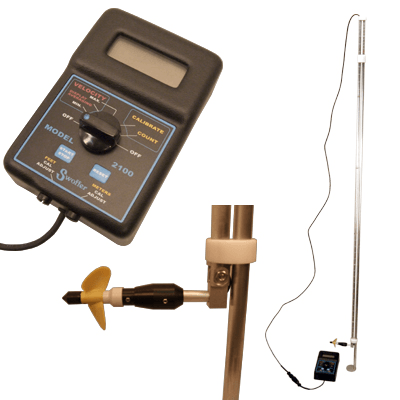In-field velocity meter. Image Credit: Wildlife Supply Company

### True Mass Meters

True mass flow meters directly measure the mass rate of flow. They include thermal meters and Coriolis meters. Thermal meters use a heated sensing element isolated from the medium flow path. The flow stream conducts heat from the sensing element, which works in conjunction with volumetric liquid flow switches. The conducted heat is directly proportional to the mass flow rate. Typically, thermal meters are used for gas flow rates. With Coriolis meters, fluid is passed through a small, vibrating flow tube. The resulting deflection of the flow tube is proportional to the mass flow rate of material.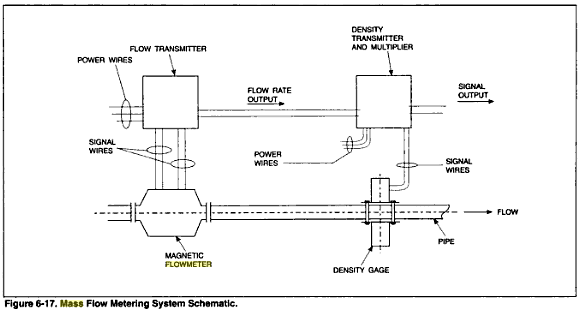Mass flow metering system schematic. Image Credit

## Specifications

Product specifications for volumetric gas flow switches include

Gas volumetric flow rate range - Gas volumetric flow rate range is the range of flow in volume/time. This specification applies to volumetric flow sensors and meters for liquids.

Operating temperature - Operating temperature is the maximum temperature that can be monitored and the average temperature of the ambient air surrounding the process. It should be considered when selecting the construction and liner materials used for the flow switch.

Operating pressure - The operating pressure is the maximum head pressure of the process media that the device can withstand. This factor should be considered when selecting the material of the flow switch.

## Resources